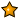# Thi vẽ biểu đồ có thưởng: chào mừng Sinh nhật GPE lần 3

##### Thành viên mớiDear Ptm0412,

I have seen that you have used this formula to change a 2D range into 1D Range:
Q2 = INDEX(X,INT((P2-0.01)/2+1),P2-INT((P2-0.01)/2+1)*2+2)
(in the clock chart).
It is a little difficult to me, but I could try to understand. The problem is that I want another formula to fill down, through all the gaps between the data's rows.

Within the clock chart in post # 39, I can do with the column P, the formula is:
P2 = 1
P3 = IF(AND(P2<>0,MOD(P2,2)=0),0,IF(P2=0,P1+1,P2+1))
then fill down.

It is OK. But when I try Q2:

Q2 = IF(P2>0,INDEX(X,INT((P2-0.01)/2+1),P2-INT((P2-0.01)/2+1)*2+2),0)
or:
Q2 = IF(P2>0,INDEX(X,INT((P2-0.01)/2+1),P2-INT((P2-0.01)/2+1)*2+2),"")
or
Q2 = IF(P2>0,INDEX(X,INT((P2-0.01)/2+1),P2-INT((P2-0.01)/2+1)*2+2),NA())

Then fill down, the chart came to the wrong way.
Now I want a formula so that I can fill down, and the chart remain its performance. Or at least, is there any way to do faster than copy and paste each time 4 cells up to over 240 cells?

Lần chỉnh sửa cuối:

#### ptm0412

Thành viên BQT
Super Moderator
Now I want a formula so that I can fill down, and the chart remains its performance.
As I know, all values such as zero length text, zero, #N/A, ... would make the chart types Line, XY Scatter perform by Excel's way: It would connect two point through the point with that kind of value.
#N/A would take effect only on Chart Type Column or Bar: No Column nor Bar appears at that point of serie.

So, my answer is noway to make any formula as you desired.

Or at least, is there any way to do faster

There are several way to do faster than copying and pasting:

1. First, use your formula with If conditon, which returns "", 0, or NA()

2. Use Autofilter within the Data area, filter zero, zero length text, or #N/A, then delete

3. For #N/A, use command Edit - Go To - Special or press Ctrl + G then press Special to select error cells only, then delete.
See this picture:#### File đính kèm

Lần chỉnh sửa cuối:

#### BNTT

##### Bùi Nguyễn Triệu Tường
Thành viên danh dự
Secondly, I would like to say thank to Mr. ptm0412, who help me a lot to know how to draw a clock by Excel chart. (what does Cheettit means?)

"Cheettit" is the pronunciation of "chết tiệt" in Vietnamese. Just only Vietnamese person could understand exactly, so it can't be translated...

Lần chỉnh sửa cuối:

##### Thành viên mớiDear ptm0412,
Such a simple way!

Dear BNTT,
Thanks for your reply, I have try to translate "chết tiệt" by google tool, but I still can't understand it.

P/S

Your English is very well, I have seen your English -Vietnamese translation of Excel_Function_Formulas and Excel Hack